Homework Help Question & Answers

# What capacitor in series with a 100 Ω resistor and a 19 mH inductor will give...

What capacitor in series with a 100 Ω resistor and a 19 mH inductor will give a resonance frequency of 1000 Hz ? Express your answer in microfarads.

#### Homework Answers

Answer #1

Resonance frequency, f = 1/ 2*pi*sqrt(L*C)

sqaring both sides,

f2=1/4 *pi*pi*L*C

C=1/4 *pi*pi*L*f2

C=1/4*3.14*3.14*19*10-3*1000*1000

C= 1.33*10-6 Farad

C=1.33 micro farad

Know the answer?
Your Answer:

#### Post as a guest

Your Name:

What's your source?

#### Earn Coin

Coins can be redeemed for fabulous gifts.

Not the answer you're looking for? Ask your own homework help question. Our experts will answer your question WITHIN MINUTES for Free.
Similar Homework Help Questions
• ### What capacitor in series with a 100 ? resistor and a 26 mH inductor will give...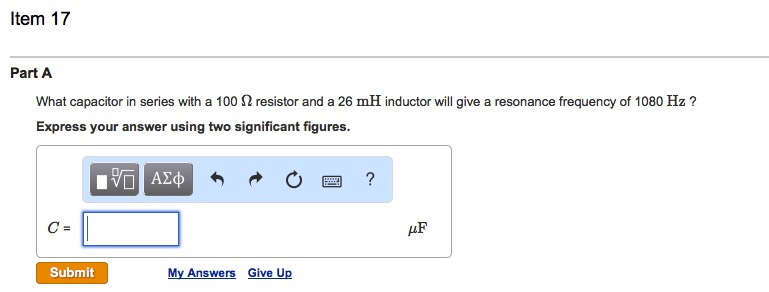What capacitor in series with a 100 ? resistor and a 26 mH inductor will give a resonance frequency of 1080 Hz ? Express your answer using two significant figures. Item 17 Part A What capacitor in series with a 100 Ω resistor and a 26 mH inductor will give a resonance frequency of 1080 Hz ? Express your answer using two significant figures. HF Submit My Answers Give Up

• ### What capacitor in series with a 100 ? resistor and a 30 mH inductor will give...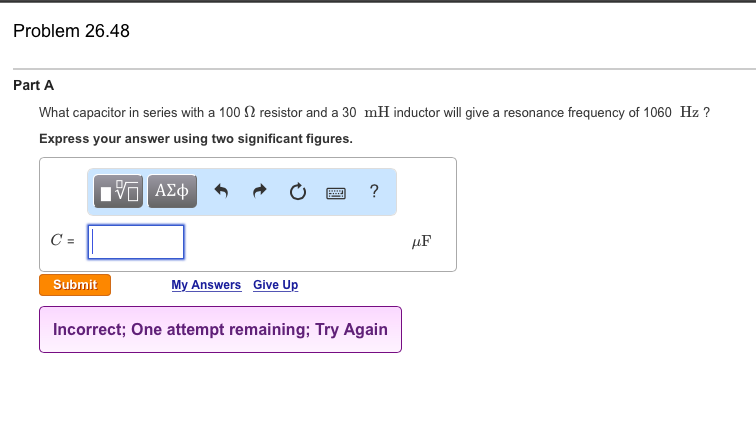What capacitor in series with a 100 ? resistor and a 30 mH inductor will give a resonance frequency of 1060 Hz ? Problem 26.48 Part A What capacitor in series with a 100 Ω resistor and a 30 mH inductor will give a resonance frequency of 1060 Hz ? Express your answer using two significant figures LF Submit My Answers Give Up Incorrect; One attempt remaining, Try Again

• ### What capacitor in series with a 100 Ω resistor and a 30 mH inductor will give...

What capacitor in series with a 100 Ω resistor and a 30 mH inductor will give a resonance frequency of 970 Hz ?

• ### What capacitor in series with a 1002 resistor and a 24.0 mH inductor will give a...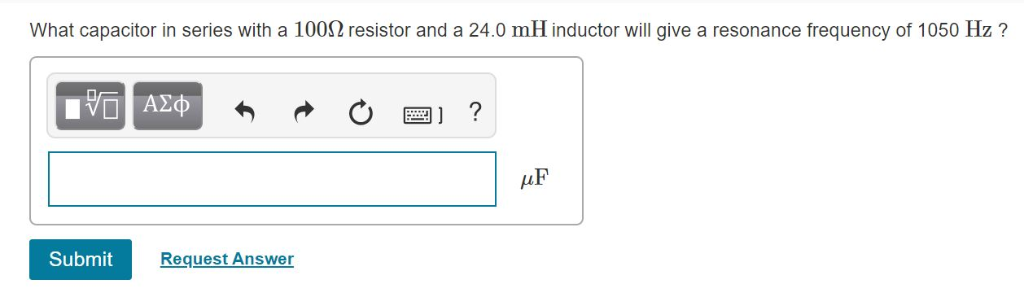What capacitor in series with a 1002 resistor and a 24.0 mH inductor will give a resonance frequency of 1050 Hz? Πνα ΑΣφ ? Submit Request Answer

• ### What capacitor in series with a 100Ω resistor and a 13.0 mH inductor will give a...

What capacitor in series with a 100Ω resistor and a 13.0 mH inductor will give a resonance frequency of 1010 Hz ?

• ### Part A What capacitor in series with a 100 resistor and a 27.0 mH inductor will...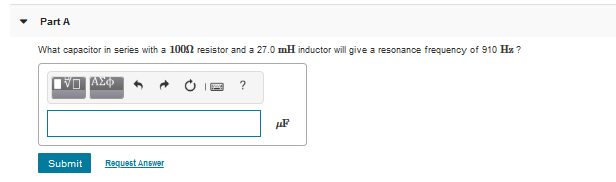Part A What capacitor in series with a 100 resistor and a 27.0 mH inductor will give a resonance frequency of 910 Hx? 1V0 AX OP? Submit Request Answer

• ### What capacitor in series with a 100 Ohm resistor and a 20.0 mH inductor will give...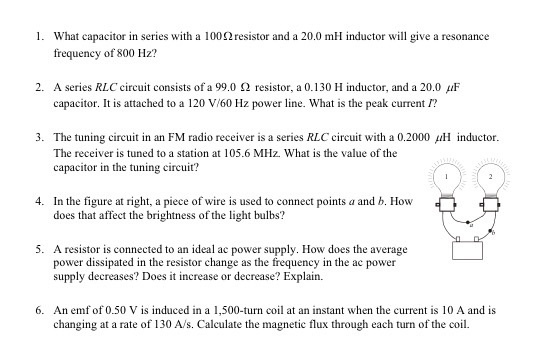What capacitor in series with a 100 Ohm resistor and a 20.0 mH inductor will give a resonance frequency of 800 Hz? A series RLC circuit consists of a 99.0 Ohm resistor, a 0.130 H inductor, and a 20.0 muF capacitor. It is attached to a 120 V/60 Hz power line. What is the peak current I? The tuning circuit in an FM radio receiver is a series RLC circuit with a 0.2000 muH inductor. The receiver is tuned to...

• ### A series RCL circuit contains a 25.8-Ω resistor, a 1.02-μF capacitor, and a 2.72-mH inductor. When...

A series RCL circuit contains a 25.8-Ω resistor, a 1.02-μF capacitor, and a 2.72-mH inductor. When the frequency is 2800 Hz, what is the power factor of the circuit?

• ### A 13 Ω resistor is connected in series with a 360 mH inductor and a 1.6...

A 13 Ω resistor is connected in series with a 360 mH inductor and a 1.6 µF capacitor. The applied voltage has the form V = V0 sin(2πf t), with voltage amplitude V0 = 497 V and frequency f = 1722 Hz . Find the effective voltage Vrms across the capacitor. Answer in units of V.

• ### 24.65 A 115-Ω resistor, a 58.5-mH inductor, and a 197-μF capacitor are connected in series to...

24.65 A 115-Ω resistor, a 58.5-mH inductor, and a 197-μF capacitor are connected in series to an ac generator. You may want to review (Pages 868 - 872) . At what frequency will the current in the circuit be a maximum? At what frequency will the impedance of the circuit be a minimum?